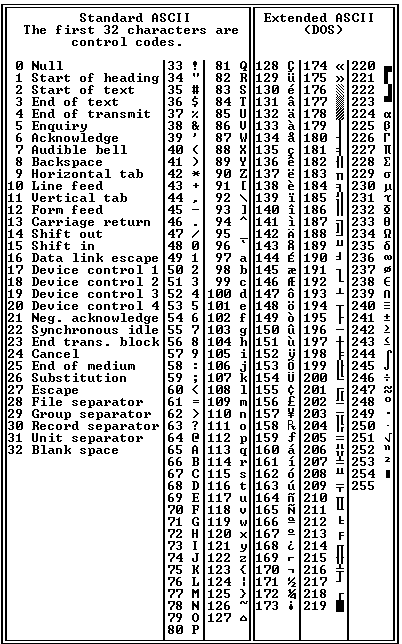# ASCII

Also found in: Dictionary, Thesaurus, Medical, Legal, Financial, Acronyms, Wikipedia.
Related to ASCII: Unicode, ASCII art

or

## American Standard Code for Information Interchange,

a set of codes used to represent letters, numbers, a few symbols, and control characters. Originally designed for teletype operations, it has found wide application in computerscomputer,
device capable of performing a series of arithmetic or logical operations. A computer is distinguished from a calculating machine, such as an electronic calculator, by being able to store a computer program (so that it can repeat its operations and make logical
. A seven-digit (or seven-bit) binary number (see binary systembinary system,
numeration system based on powers of 2, in contrast to the familiar decimal system, which is based on powers of 10. In the binary system, only the digits 0 and 1 are used.
) can represent one of 128 distinct codes. Thus, in decimal equivalents, the series "72, 69, 76, 76, 79" represents the letters "h, e, l, l, o" in ASCII. With the introduction of its personal computer in 1981, the International Business Machines Company (IBM) increased the number of available characters to 256 by using an eight-bit byte. This IBM-extended ASCII set has become a de facto standard. However, the inability of US-ASCII to correctly represent many other languages became an obvious and intolerable misfeature as computer use outside the United States and United Kingdom increased. As a consequence, national extensions to US-ASCII were developed that were incompatible with one another. This in turn led to the standardization of 16-bit (or "double-byte") and 32-byte character sets, such as UnicodeUnicode
, set of codes used to represent letters, numbers, control characters, and the like, designed for use internationally in computers. It has been expanded to include such items as scientific, mathematical, and technical symbols, and even musical notation.
, that could accommodate large numbers of linguistic and other symbols.

## ASCII

[′as‚kē]
(communications)
McGraw-Hill Dictionary of Scientific & Technical Terms, 6E, Copyright © 2003 by The McGraw-Hill Companies, Inc.

## ASCII

American standard code for information interchange: a computer code for representing alphanumeric characters
Collins Discovery Encyclopedia, 1st edition © HarperCollins Publishers 2005

## ASCII

(American Standard Code for Information Interchange) Pronounced "ask-ee," ASCII is the built-in binary code for representing characters in all computers except IBM mainframes, which use EBCDIC coding. ASCII was originally developed for communications and uses seven bits per character, providing 128 combinations that include upper and lower case alphabetic letters, the numeric digits and special symbols such as the \$ and %. The first 32 characters are format codes (tab, return, etc.) as well as control codes for communications and printers (see below). See ASCII file, ASCII ribbon campaign, EBCDIC and Unicode.

ASCII vs. Hex
In technical editors used by developers, there is a choice between entering data in ASCII or hexadecimal ("hex"). ASCII is all characters, but hex is limited (0 to 9 and A to F). See hex chart and hex editor.

A Byte Holds ASCII and Then SomeThe common storage unit in a computer is an 8-bit byte that holds 256 character combinations (0-255). However, ASCII uses only the first 128 (0-127), and the rest (128-255) are foreign language and math symbols.
Copyright © 1981-2019 by The Computer Language Company Inc. All Rights reserved. THIS DEFINITION IS FOR PERSONAL USE ONLY. All other reproduction is strictly prohibited without permission from the publisher.
References in periodicals archive ?
a) [MATHEMATICAL EXPRESSION NOT REPRODUCIBLE IN ASCII.], (5.1) where [MATHEMATICAL EXPRESSION NOT REPRODUCIBLE IN ASCII.]
where [w.sub.s] is continuous and [MATHEMATICAL EXPRESSION NOT REPRODUCIBLE IN ASCII.].
Because X is Ito-differentiable we can write [MATHEMATICAL EXPRESSION NOT REPRODUCIBLE IN ASCII.] (Lemma 4.1) and (5.3) becomes equivalent to
Let X: [infinity] x [OMEGA] [right arrow] [R.sup.n] be a differentiable stochastic process, a: [0, [infinity]) x [R.sup.n] [right arrow] R continuous, [MATHEMATICAL EXPRESSION NOT REPRODUCIBLE IN ASCII.].
If [MATHEMATICAL EXPRESSION NOT REPRODUCIBLE IN ASCII.], then [MATHEMATICAL EXPRESSION NOT REPRODUCIBLE IN ASCII.]
Ito formula allows us to find [MATHEMATICAL EXPRESSION NOT REPRODUCIBLE IN ASCII.] an then applying (3.5) we get the [sigma]-equality.

Site: Follow: Share:
Open / Close